# Memoryless channel

(diff) ← Older revision | Latest revision (diff) | Newer revision → (diff)

A communication channel for which the statistical properties of the output signal at a timeare determined only by the input signal transmitted at this momentof time (and consequently do not depend on the signal transmitted prior to or after the moment). More precisely, a discrete-time communication channel whose input and output signals are given by random sequencesandwith values in spaces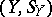and, respectively, is called a memoryless channel if for any natural numberand any sets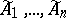,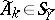,, the equality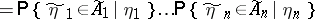holds, where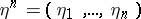. If furthermore the conditional probabilities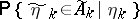do not depend on, then the channel is called a homogeneous memoryless channel.

If one denotes by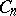the transmission rate of the channel (cf. Transmission rate of a channel) for a segment of lengthof a homogeneous memoryless channel, then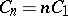. Ifandare finite (or countable) sets, a homogeneous memoryless channel is completely determined by the matrix of transition probabilities, where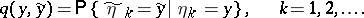For references see , – cited in Communication channel.

How to Cite This Entry:
Memoryless channel. Encyclopedia of Mathematics. URL: http://encyclopediaofmath.org/index.php?title=Memoryless_channel&oldid=16884
This article was adapted from an original article by R.L. DobrushinV.V. Prelov (originator), which appeared in Encyclopedia of Mathematics - ISBN 1402006098. See original article